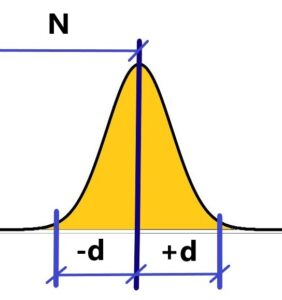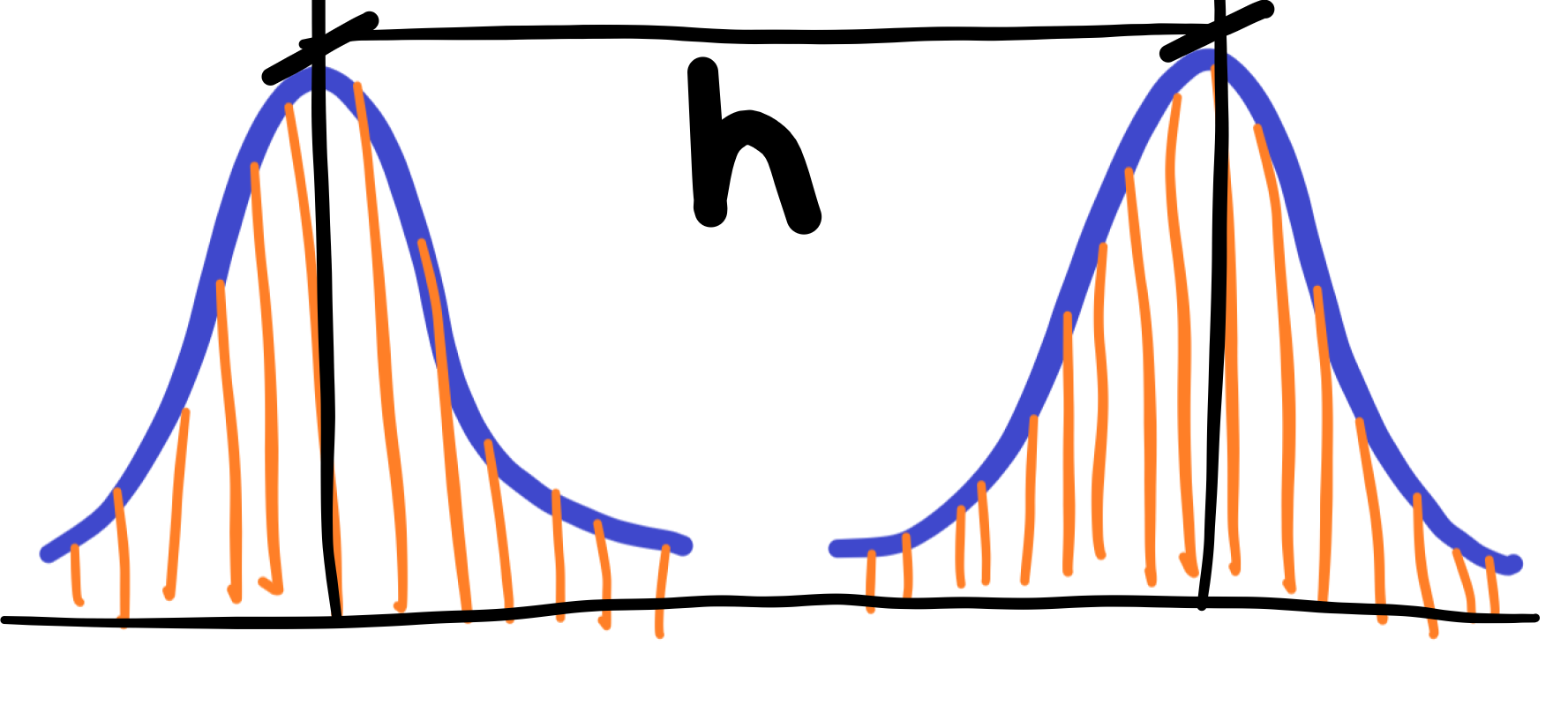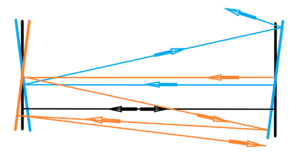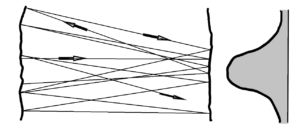Where is the right value N? We are unable to measure directly right value N of something – diameters of balls or anything else. We are only able to measure a certain amount of values that, in effect, when plotted, resemble Gauss’s distribution curve of random errors. In other words, we consider the peak of this curve to be the right value N.Measured values oscillate at interval N ± d over the right value N, which is irrational (see rational numbers oscillate around irrational ones to infinity) and, crucially, the indescribable right value N keep changing – it’s “alive”.
The prove – t
he Gaussian distribution curve also oscillates, slightly, through the time, when we take several sets of measurements of the value N – e.g. a diameter of a ball. We could say “Probability of probabilities”.

Let´s go to the emission of light. E.g. the wavelenght N of emitted light from hydrogen atom. Specifically, the line spectra, so characteristic of each element from hydrogen, helium, through sodium, etc. The line spectrum needs to be taken realistically and not strictly mathematically. There is no ideal line without thickness in the world, just as there is no ideal point or ideal circle. Reality, then, is always a bit of a blur. It follows that our measurements are inaccurate. Nor is there an absolutely sharp scratch of the measuring instrument. Thus, even the so-called sharp line spectrum of emitted light has a very small yet fuzzy nature. It is best expressed again by the Gaussian distribution curve.

We will never measure two identical values at the wavelength level of light – see orange lines in an image below. Light is part of an ocean of quantum field full of random quantum fluctuations. Never repeatable. The idea for theory of dissipation or irreversibility.We take the distance between two peaks h of the two curves for the value of the “correct”  wavelength. It should be added that there is no exact value of the wavelength of light emitted, for this nature – a kind of blurism is inherent in the nature of real nature in and around us. So it would be better to no longer call this attribute, in the form of a mathematical notation and graph, the distribution curve of measurement errors. However, this is a natural characteristic of real nature. This is always alive and changing Life! We also don’t call the error the movement of molecules or we don’t call the error the range of the oscillating movement of the spring. Would we then call the movement of an elephant or a human in a restricted place the error? However, it is meant to sit in the middle and not walk at different distances from chair to room? Or shall we call pregnancy a disease?

Go back to probability distribution curve of orrurence – can sometimes help us. See the construction of lasers or interferometers – a resonant tube between two mirrors. We need two mirrors that are parallel to each other – planparallel. Further, these mirrors must be as planar as possible – to a tenth of the wavelength of sodium light. But too much of anything is bad for them. Too much flatness could be a disadvantage. We can’t exactly set two mirrors exactly parallel to each other. There’s always going to be some deviation. The point is to keep them as small as possible. If the two mirrors were ideally planar, we would miss the light ray from the resonant tube before it could excite the other electron levels to emit light in phase with the excitation light ray – coherence of light. See the below figure on the left. The rays waveforms: black for the ideal state, which cannot exist, and the other two waveforms (blue and orange) for very real deviations in the relative positions of the mirrors. We see that the light ray leaves the resonant tube between the mirrors very soon.In the second figure above we see the irregularities in the flatness of the two mirrors – the randomly wavy surface – the roughness of the glass surface. And it is immediately clear that the light ray travels randomly in different directions, but most of the light rays remain in the resonant tube between the mirrors for the required time in accordance of the curve of probability distribution. And thus the problem is satisfied for the right operation of the laser or interferometer.

Resolution versus regulation. It’s impossible to regulate processes that we can’t distinguish. For regulation, we need a distinguishable impulse. The more precise the scale, the more precise the regulation. However, there is some regulatory limit, or limit of distinguishability, not only for optical instruments in terms of diffraction, or the thermal movement of molecules, but above all the fundamental law of quantum mechanics – the Heisenberg uncertainty principle.